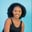Related Tags

numpy
python3

# How to use the numpy.cbrt() method for a 2-D array in PythonMaria Elijah

## The numpy.cbrt() method

The numpy.cbrt() function calculates the cube root of an input array. This is done element by element.

Note: In Python, we can use a list of lists to create a two-dimensional (2-D) array.

### Syntax

numpy.cbrt(x,out=None, where=True)


### Parameters

• x: This specifies the input array.
• out: This is an optional parameter. It represents the location where the result is stored.
• where: This is an optional parameter. It represents the condition in which the input gets broadcasted.

### Return value

The numpy.cbrt() method returns the cube root of an input array.

### Example

The following code shows how to use the numpy.cbrt() method for two-dimensional (2-D) arrays in Python.

# import numpy
import numpy as np
# create 2D array using np.array
x = np.array([[6,3,7,2], [8,6,9,5]])
# Compute the cube root
# using np.cbrt()
result = np.cbrt(x)

print(result)
Implementation of numpy.crbt() method

### Explanation

• Line 2: We import the library numpy.
• Lines 4–6: We create a two-dimensional array called x.
• Line 7: To calculate the cube root of the input array x, we use the np.cbrt() method.
• Line 9: We display the result.

RELATED TAGS

numpy
python3

CONTRIBUTORMaria Elijah
RELATED COURSES

View all Courses

Keep Exploring

Learn in-demand tech skills in half the time